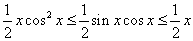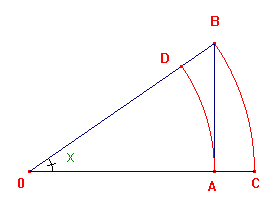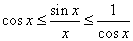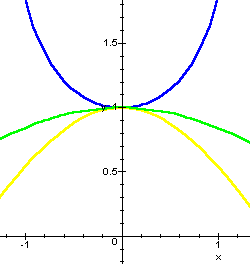www.batmath.it

The limit of sinx/x as x tends to zero

Consider a right angled triangle OAB, with hypotenuse OB=1, and BOA=x. Then the area of the circular segment OAD is less than the area of the triangle OAB which is less than that of the circular segment OCB. As OA=cosx, BA=sinx, we obtain:.If we divide by (1/2)xcosx, we obtain:.

If we change x with -x, nothing changes. So we may conclude.

The three graphs are plotted here below.first published on march 26 2002 - last updated on september 01 2003